# IB DP Physics: SL复习笔记2.3.6 Principle of Conservation of Energy

### Principle of Conservation of Energy

• The Principle of Conservation of Energy states that:

Energy cannot be created or destroyed, it can only be transferred from one form to another

• This means the total amount of energy in a closed system remains constant, although how much of each form there is may change

Types of Energy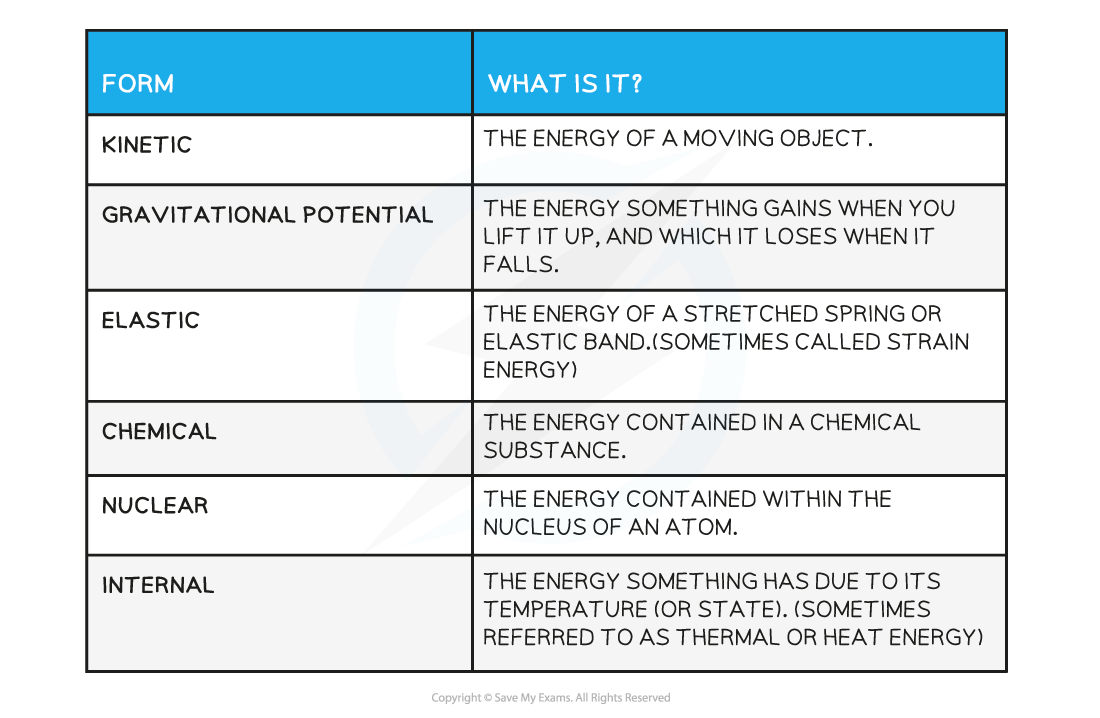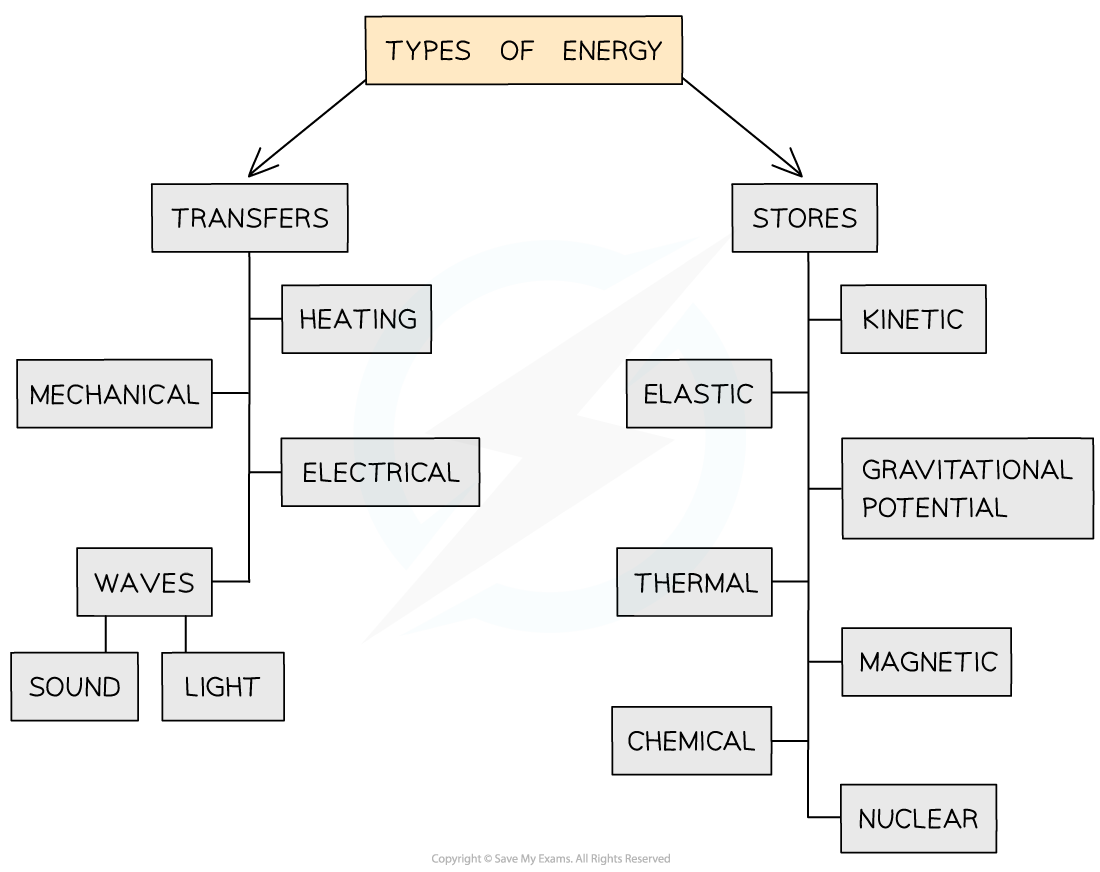Energy types can be separated into transfers or stores

• The differences between energy stores and energy transfers can be confusing, so it is important to learn their differences and the different types of energy involved in each

Energy Stores

• Energy stores keep or store energy within one part of a system
• Kinetic energy store: the amount of energy in a kinetic energy store depends on the speed of the object
• A runner with a high kinetic energy store in her muscles can run more quickly than a runner with a smaller kinetic energy store
• A car with a high kinetic energy store within the engine can drive more quickly than a car with a smaller kinetic energy store
• A racing car has a higher kinetic energy store than a tractor
• Gravitational potential energy store: the amount of energy stored in an object depending on its height
• The higher an object, the more gravitational potential energy it has
• When a diver stands on a board 5 m high he has more gravitational potential energy than when standing on a board 3 m high
• Magnetic energy store: magnets store magnetic energy until a magnetic material is present in its field
• A piece of iron will be moved when it enters a magnetic field
• The magnetic energy store is transferred to the kinetic energy of the iron
• Chemical energy store: energy is stored as chemical energy and a chemical reaction takes place to release and then transfer it
• Chemical energy is stored in our body for use when we think and move
• Chemical energy is stored in a battery to be transferred to electrical energy
• Thermal energy store: all objects store thermal energy
• An object that is hotter stores more thermal energy than an object that is colder
• Nuclear energy store: in a nuclear reactor, energy is stored as Uranium-235 until it is bombarded by neutrons and a huge amount of thermal energy is released

Energy Transfers

• Energy transfers: give or transfer energy to different parts of a system
• They act as a pathway around an energy system
• Electrical transfer: when charge flows to produce an electric current
• The current transfers the energy
• Mechanical transfer: this occurs when a force is applied to move an object
• This could be pushing a book across a desk
• It could also be sound waves passing through a material causing the particles to move
• Heating transfer: The internal energy of an object is determined by the temperature of the object
• Energy is transferred from hotter to cooler areas
• Waves transfer: When sound travels through a material it causes the particles to vibrate
• Energy is transferred from an object that is moving/vibrating to generate the sound waves through other objects by the movement of particles
• Light energy is transferred from the sun so we can see

#### Energy Dissipation

• When energy is transferred from one form to another, not all the energy will end up in the desired form (or place)
• Dissipation is used to describe ways in which energy is wasted
• Any energy not transferred to useful energy stores is wasted because it is lost to the surroundings
• These are commonly in the form of thermal (heat), light, or sound energy
• What counts as wasted energy depends on the system
• For example, in a television:

electrical energy ➝ light energy + sound energy + thermal energy

• Light and sound energy are useful energy transfers whereas thermal energy (from the heating up of wires) is wasted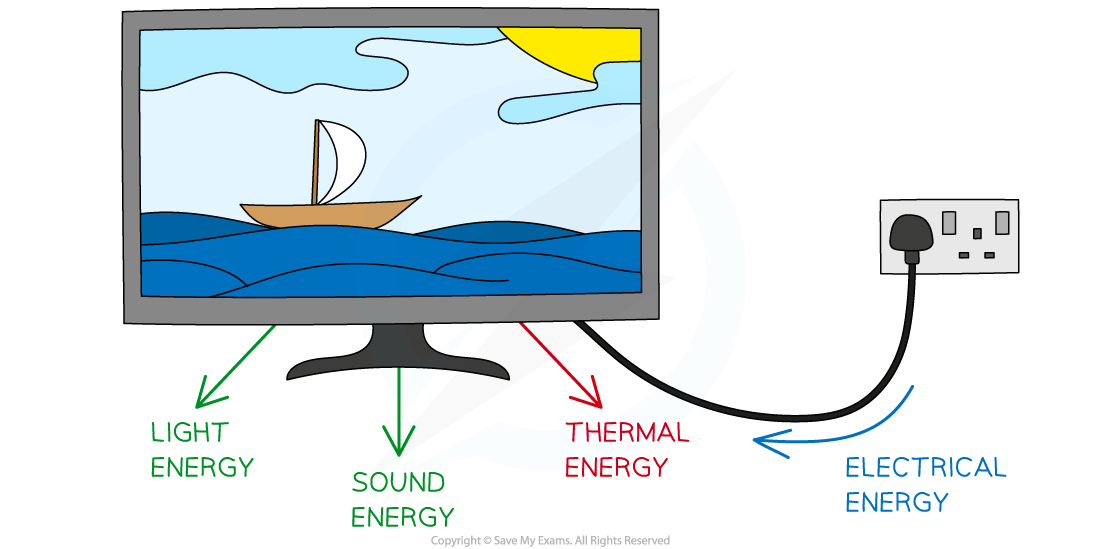Useful and wasted energy conversions for a television

• The energy changes in an electrical heater:electrical energy ➝ thermal energy + sound energy + light energy
• In a gas cooker, the energy transfers are similar but the initial source of energy is different:

chemical energy ➝ thermal energy + sound energy + light energy

• In both these cases, thermal energy is useful, whereas sound and light are not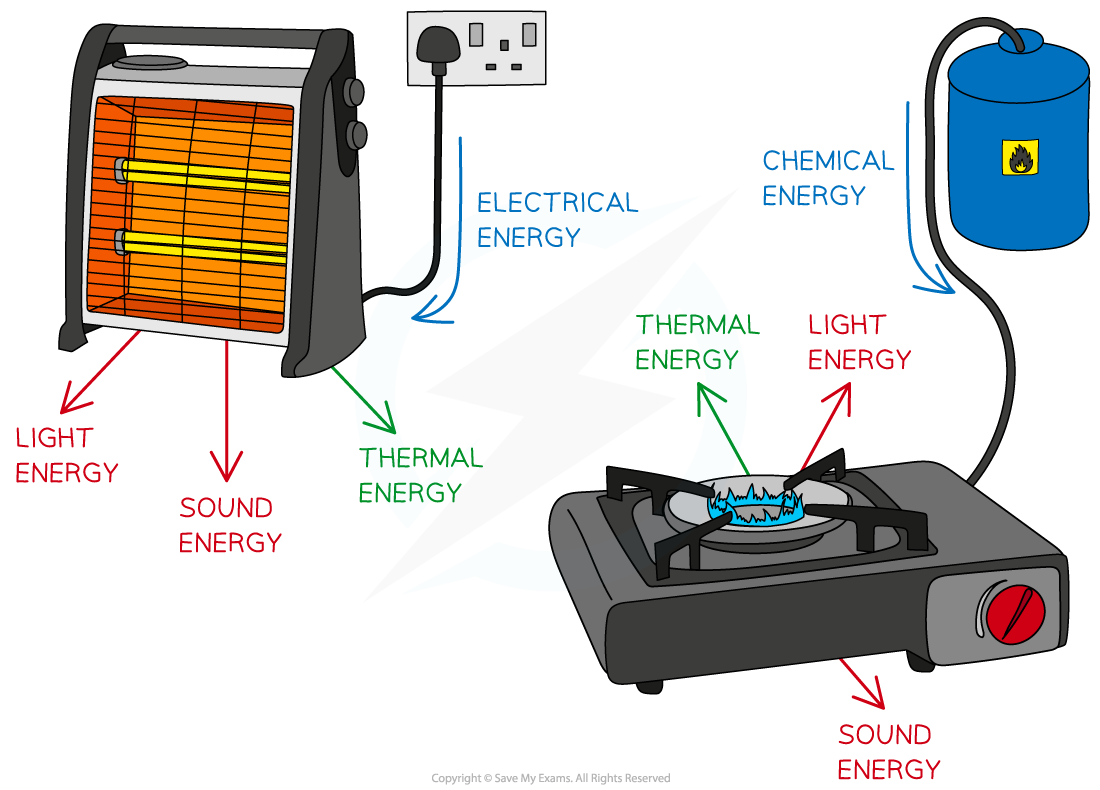Useful and wasted energy conversions in an electric heater and gas cooker

#### Worked Example

The diagram shows a rollercoaster going down a track.

The rollercoaster takes the path A → B → C → D.Which statement is true about the energy changes that occur for the rollercoaster down this track?

A.     KE - GPE - GPE - KE

B.     KE - GPE - KE - GPE

C.     GPE - KE - KE - GPE

D.     GPE - KE - GPE - KE

• At point A:
• The rollercoaster is raised above the ground, therefore it has GPE
• As it travels down the track, GPE is converted to KE and the roller coaster speeds up
• At point B:
• KE is converted to GPE as the rollercoaster rises up the loop
• At point C:
• This GPE is converted back into KE as the rollercoaster travels back down the loop
• At point D:
• The flat terrain means the rollercoaster only has KE

#### Applications of Energy Conservation

• In mechanical systems when energy is transferred between stores it is equivalent to the work done:
• A falling object (in a vacuum, where no energy is not dissipated into the surroundings): gravitational potential energy ➝ kinetic energy
• Horizontal mass on a spring: elastic potential energy ➝ kinetic energy
• We can also say energy is transferred between stores and transfers:
• A battery connected to a bulb: chemical energy ➝ electrical energy ➝ light energy (if connected to a bulb)
• A car: chemical energy ➝ mechanical energy ➝ kinetic energy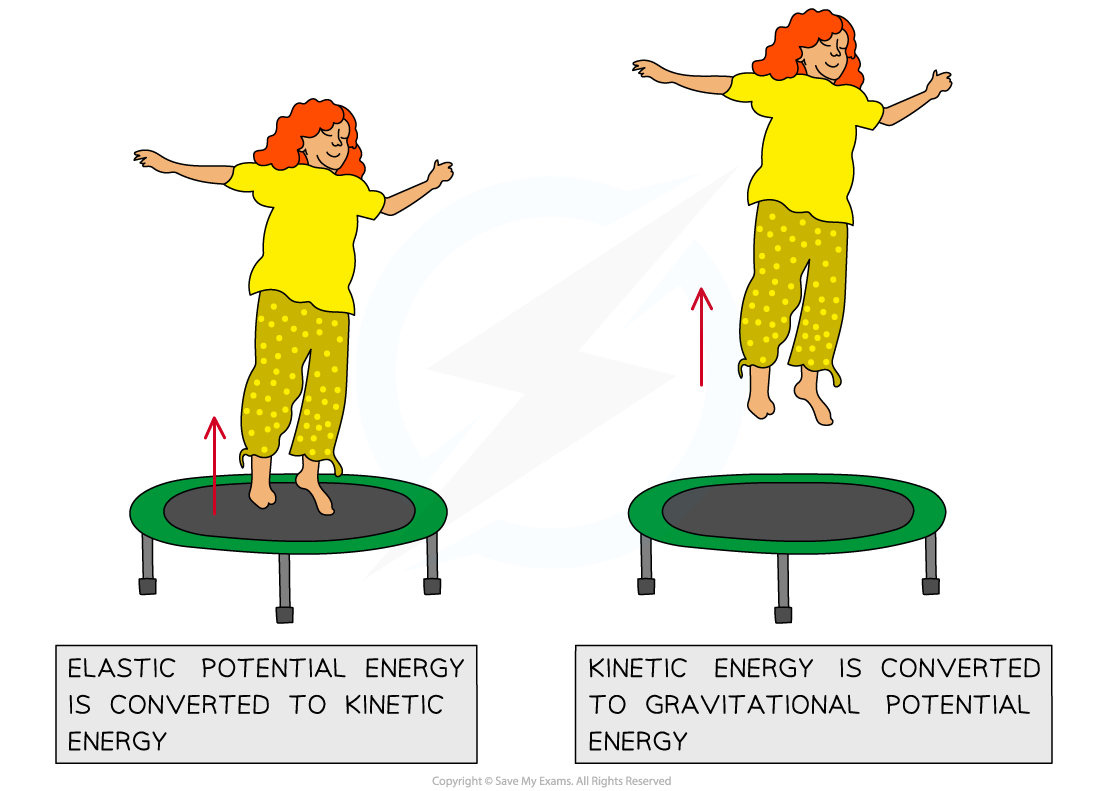Energy transfers whilst jumping on a trampoline

• There may also be work done against resistive forces such as friction
• For example, if an object travels up a rough inclined surface, then

Loss in kinetic energy = Gain in gravitational potential energy + Work done against friction

#### Worked Example

A simple pendulum has a mass of 640 g and a length of 0.7 m. It is pulled out to an angle of 20° from the vertical.The pendulum is released. Assuming negligible air resistance, calculate the maximum speed of the pendulum bob as it passes through the vertical position.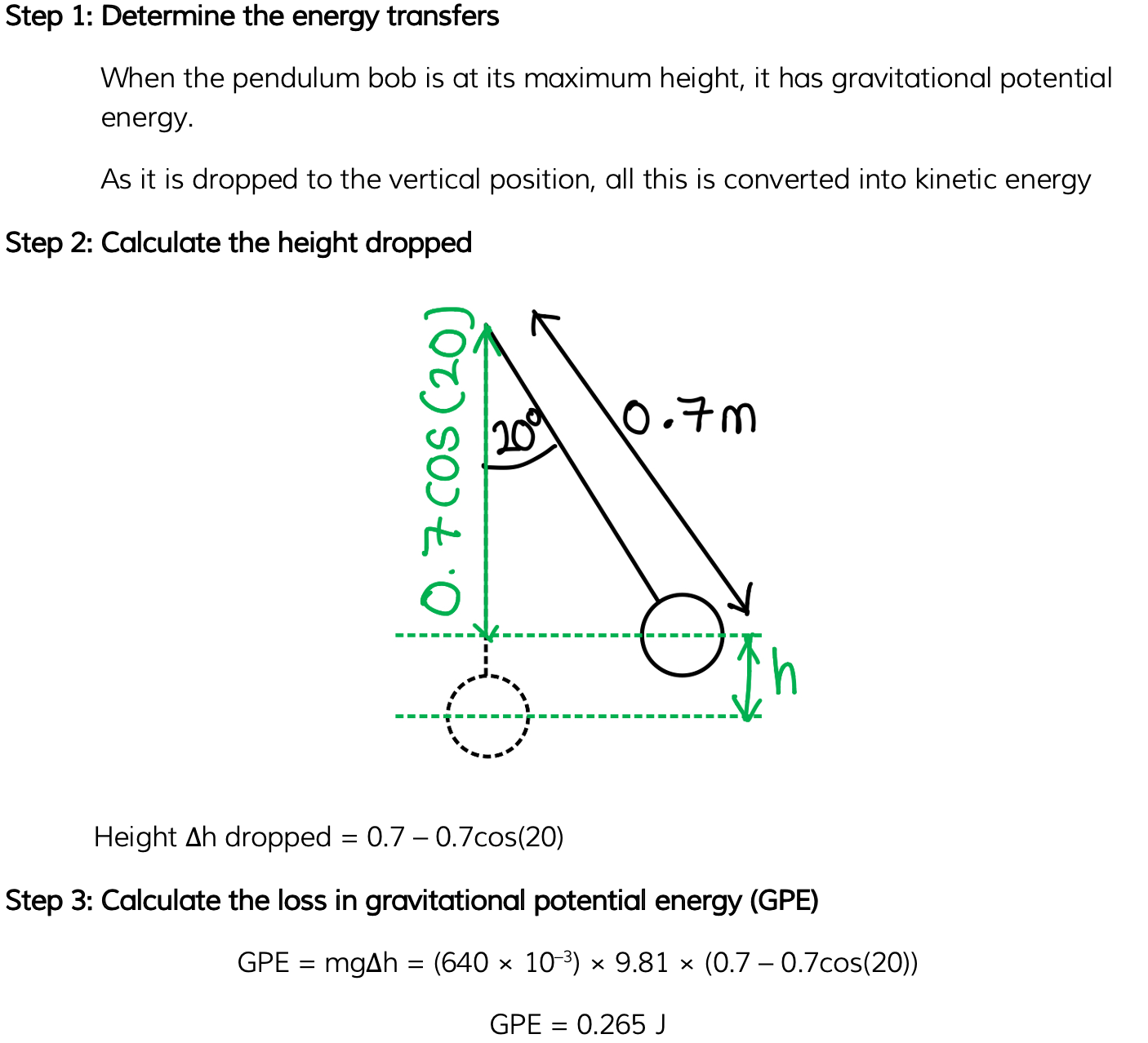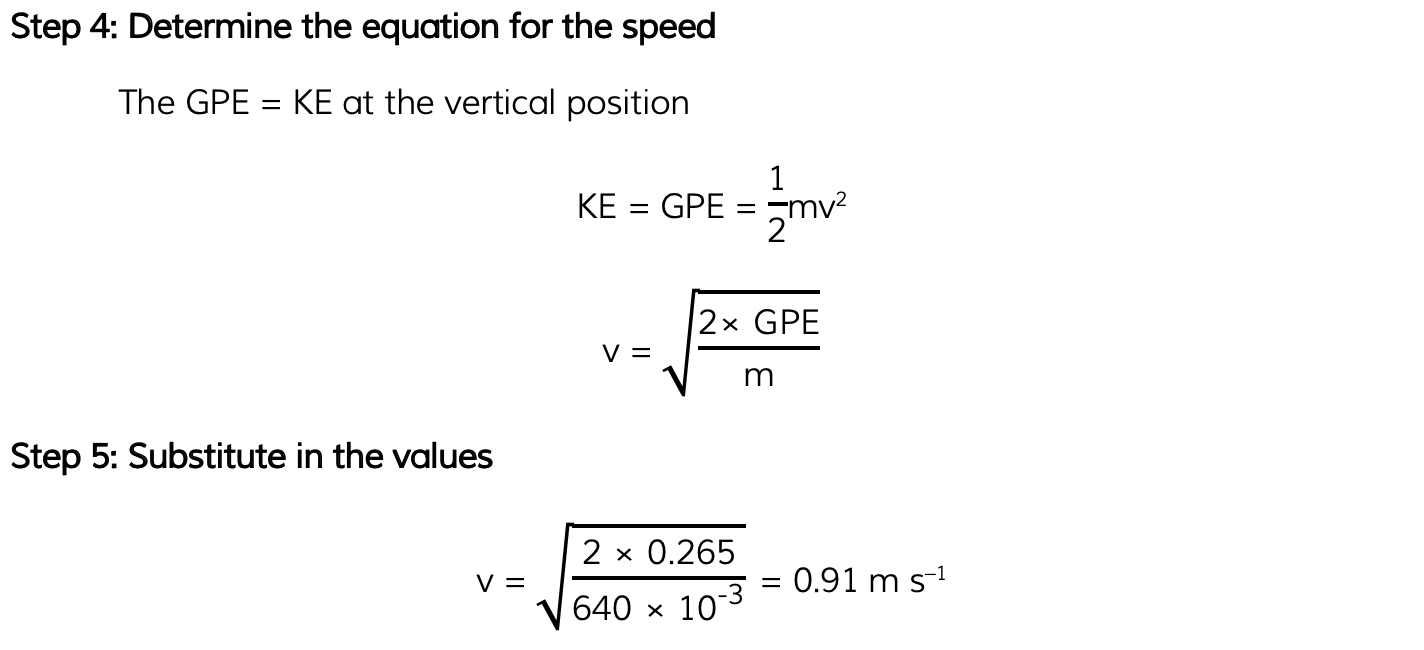#### Spring Energy Conservation

• When a vertical spring is extended and contracted, its energy is converted into other forms
• Although the total energy of the spring will remain constant, it will have changing amounts of:
• Elastic potential energy (EPE)
• Kinetic energy (KE)
• Gravitational potential energy (GPE)
• When a vertical mass is hanging on a spring and it moves up and down, its energy will convert between the three in various amounts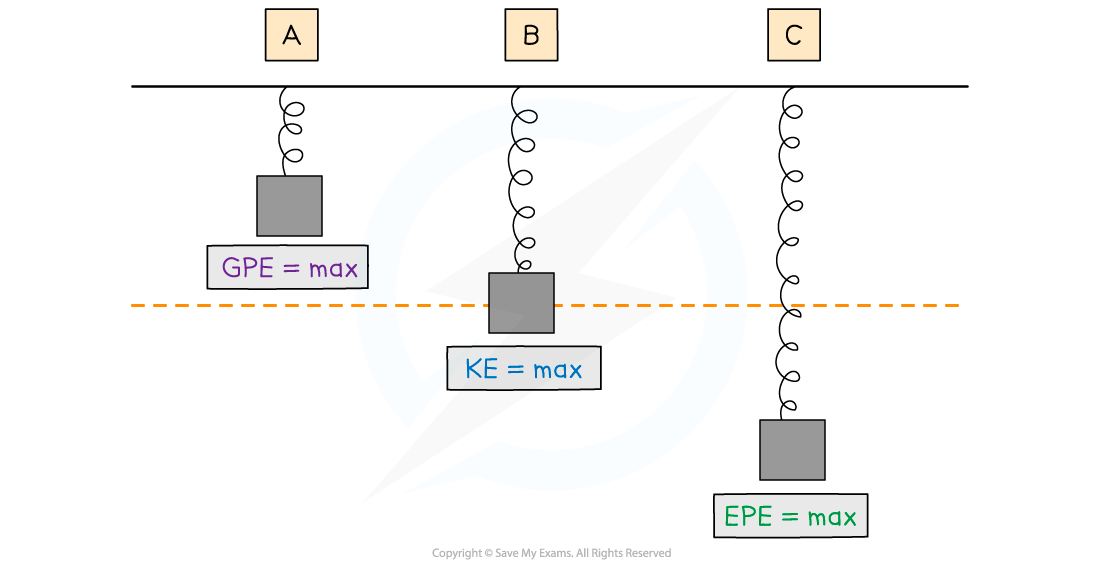• At position A:
• The spring has some EPE since it is slightly compressed
• Its KE is 0 since it is stationary
• Its GPE is at a maximum because the mass is at its highest point
• At position B:
• The spring has some EPE since it is slightly stretched
• Its KE is at a maximum as it passes through the equilibrium position at its maximum speed
• It has some GPE since the mass is still above the ground
• At position C:
• The spring has its maximum EPE because it is at its maximum extension
• Its KE is 0 since it is stationary
• Its GPE is at a minimum because it is at its lowest point above the Earth's surface
• For a horizontal mass on a spring system, there is no gravitational potential energy to consider. The spring only converts between kinetic and elastic potential energy